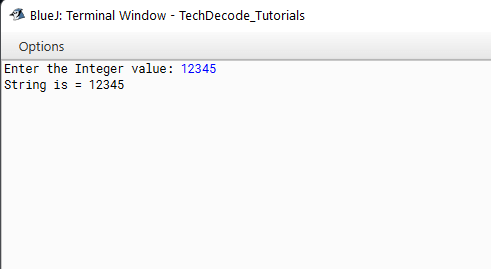# How to convert int to String in Java

We have all learned the concept of an integer in elementary school. Integers are numbers without fractional portions. In other words, integers are like whole numbers, but they also include negative values. As a result, non-decimal numbers can be both positive and negative. It’s a memory place (or locations) that stores non-decimal or non-fractional positive or negative numbers. The keyword ‘int’ denotes an integer type, which describes the memory required to store the data. This type also has limitations on how it may be used. A fractional number, for example, cannot be stored in an integer. Strings are Objects in Java that are internally maintained by a char array. Strings are immutable since arrays are immutable (they can’t expand). Each time you make an update to a String, a new String is constructed. So today let us start with How to convert Int to String in Java.

## What’s The Approach?

• Import the` java.util` package into the class.

• Now within the main method create a new object of Scanner class, for example `Scanner sc=new Scanner(System.in);`

• Use the `Scanner` class to get the input from the user.

• Implement` Integer.toString(int_variable)` to convert the string to an integer type

### Java Program to convert Int to String in Java

```/*
* TechDecode Tutorials
*
* How to Covert Integer to String
*
*/
import java.util.*;
public class Int_to_String
{
public static void main(String args[])
{
// Creating an object of Scanner class
Scanner sc= new Scanner(System.in);
// taking imput from  the user
System.out.print("Enter the Integer value: ");
int num=sc.nextInt();
// converting integer to string
String s = Integer.toString(num);
System.out.println("String is = " + s);
}
}
```

Output:-